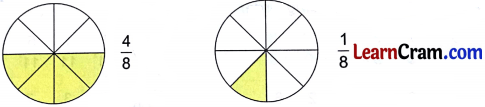# DAV Class 3 Maths Chapter 11 Worksheet 3 Solutions

The DAV Maths Book Class 3 Solutions and DAV Class 3 Maths Chapter 11 Worksheet 3 Solutions of Fractions offer comprehensive answers to textbook questions.

## DAV Class 3 Maths Ch 11 WS 3 Solutions

Question 1.
Use the symbol ‘>’ or ‘<‘ at proper places.

(a) $$\frac{2}{6}$$ ___ $$\frac{5}{6}$$
Solution:
<

(b) $$\frac{7}{8}$$ ___ $$\frac{9}{8}$$
Solution:
<

(c) $$\frac{5}{11}$$ ___ $$\frac{3}{11}$$
Solution:
>

(d) $$\frac{7}{3}$$ ___ $$\frac{5}{3}$$
Solution:
>

(e) $$\frac{6}{4}$$ ___ $$\frac{7}{4}$$
Solution:
<

(f) $$\frac{11}{9}$$ ___ $$\frac{13}{9}$$
Solution:
<Question 2.
Encircle the fraction which is smaller in each. The first one is done for you.

(a) $$\frac{1}{5}$$, $$\frac{4}{5}$$
Solution:
$$\frac{1}{5}$$

(b) $$\frac{5}{6}$$, $$\frac{3}{6}$$
Solution:
$$\frac{3}{6}$$

(c) $$\frac{2}{3}$$, $$\frac{1}{3}$$
Solution:
$$\frac{1}{3}$$

(d) $$\frac{1}{4}$$, $$\frac{3}{4}$$
Solution:
$$\frac{1}{4}$$

(e) $$\frac{2}{5}$$, $$\frac{4}{5}$$
Solution:
$$\frac{2}{5}$$

(f) $$\frac{2}{8}$$, $$\frac{5}{8}$$
Solution:
$$\frac{2}{8}$$Question 3.
Arrange in ascending order.

(a) $$\frac{7}{9}, \frac{3}{9}, \frac{5}{9}, \frac{11}{9}, \frac{8}{9}$$
Solution:
$$\frac{3}{9}<\frac{5}{9}<\frac{7}{9}<\frac{8}{9}<\frac{11}{9}$$

(b) $$\frac{7}{11}, \frac{1}{11}, \frac{5}{11}, \frac{4}{11}, \frac{9}{11}$$
Solution:
$$\frac{1}{11}<\frac{4}{11}<\frac{5}{11}<\frac{7}{11}<\frac{9}{11}$$

(c) $$\frac{3}{5}, \frac{4}{5}, \frac{1}{5}, \frac{2}{5}, \frac{6}{5}$$
Solution:
$$\frac{1}{5}<\frac{2}{5}<\frac{3}{5}<\frac{4}{5}<\frac{6}{5}$$

(d) $$\frac{8}{10}, \frac{3}{10}, \frac{7}{10}, \frac{6}{10}, \frac{1}{10}$$
Solution:
$$\frac{1}{10}<\frac{3}{10}<\frac{6}{10}<\frac{7}{10}<\frac{8}{10}$$Question 4.
Arrange in descending order.

(a) $$\frac{3}{6}, \frac{1}{6}, \frac{5}{6}, \frac{11}{6}, \frac{2}{6}$$
Solution:
$$\frac{11}{6}>\frac{5}{6}>\frac{3}{6}>\frac{2}{6}>\frac{1}{6}$$

(a) $$\frac{1}{8}, \frac{7}{8}, \frac{6}{8}, \frac{5}{8}, \frac{4}{8}$$
Solution:
$$\frac{7}{8}>\frac{6}{8}>\frac{5}{8}>\frac{4}{8}>\frac{1}{8}$$

(a) $$\frac{4}{7}, \frac{2}{7}, \frac{9}{7}, \frac{1}{7}, \frac{10}{7}$$
Solution:
$$\frac{10}{7}>\frac{9}{7}>\frac{4}{7}>\frac{2}{7}>\frac{1}{7}$$

(a) $$\frac{10}{12}, \frac{5}{12}, \frac{11}{12}, \frac{1}{12}, \frac{3}{12}$$
Solution:
$$\frac{11}{12}>\frac{10}{12}>\frac{5}{12}>\frac{3}{12}>\frac{1}{12}$$### DAV Class 3 Maths Chapter 11 Worksheet 3 Notes$$\frac{4}{8}$$ > $$\frac{1}{8}$$

If the fractions have same denominator then fraction having greater numerator represents the greater fraction.